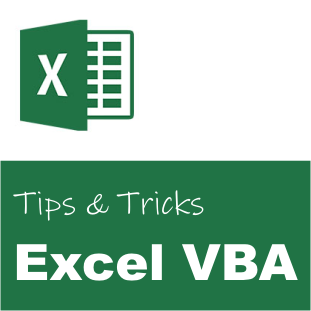# Excel VBA: Custom SumIF function that ignores N/A# Excel VBA: Custom SumIF function that ignores N/A

Below we have made a custom function which sums the values of one column/range given that the value of another column is equal to a fixed value. This function ignores the N/A values, which is not the case for the built-in function called SumIf in Excel. If the built-in function finds a N/A value the function returns N/A.

```' SUM Value If
' Ignores N/A
Public Function SumValueIf(ByVal objRange As Range, ByVal objCriteria As Range, ByVal objSumRange As Range) As Currency
Dim intRow As Integer
Dim objRangeValue As Object
Dim objCriteriaValue As Object
Dim objValue As Object
Dim dblValue As Currency
Dim dblSum As Currency

' Get the criteria value
objCriteriaValue = objCriteria(1, 1)

' Walk through the rows
For intRow = 1 To objRange.Rows.Count Step 1
' Get the current value
objRangeValue = objRange(intRow, 1)

' Compare values
If (objRangeValue = objCriteriaValue) Then
' Get the value
objValue = objSumRange(intRow, 1)

' Is valid number?
' Ignore any strings, #N/A, #Error, etc.
If (IsNumeric(objValue)) Then
' Get the value
dblValue = CCur(objValue)

' Sum
dblSum = dblSum + dblValue
End If
End If
Next

' Get the value
SumValueIf = dblSum
End Function```

The function can be used in the following way in a Excel cell:

= SumValueIf(A1:A10; “abc”; B1:B10)

where A1:A10 is the compare column. Only sum the value in column B1:B10 if the value in column A is equal to “abc”.

### Related##### Ulf Emsoy
Ulf Emsoy has long working experience in project management, software development and supply chain management.